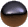Reading Your Gas MeterBasic CalculationsPressure Meter CalculationsConvert SCFH to BtuH or BHConvert BtuH or BH to SCFHReturn to Norgasco Home

Example:

23,456 - 12,345 = 11,111, so the difference is actually 1,111,100 cubic feet (note that this should not be confused with standard cubic feet (SCF).

To get SCF from the meter reading there are two meter factors normally used - pressure meter factor and temperature meter factor. Norgasco's meters in Deadhorse automatically correct for temperature so the only meter factor we are concerned with is the pressure meter factor. (return to directory)

Pressure Meter Calculations

(14.65 (meter atmospheric pressure) + Gauge pressure of the meter) 14.73 (standard atmospheric pressure)

so if, in the example above, your meter pressure is 30 psi your meter factor would be (14.65+30) ¸ 14.73 = 3.0312

1,111,100 x 3.0312 = 3,367,966.3 SCF

Now, to calculate the number of MMBtu's for the period in question, use the current heating value (usually around 950 Btu/SCF).

Using 950 Btu/SCF for our example,

3,367,966.3 x 950 = 3,199,567,985 Btu's

divide this number by 1,000,000 to obtain MMBtu's

To get BtuH or BH (Btu's per hour) from SCFH (standard cubic feet per hour)

Example:

Using the American meter catalog, you see that the meter has a capacity of 25,000 SCFH at 30 psi for .6 specific gravity gas.

Our natural gas has a specific gravity of .73. From NFPA 54 the conversion factor is .9 so 25,000 SCFH x .9 = 22,500 SCFH

Design Btu/Cubic foot is 940 so 22,500 SCFH x 940 Btu/CF = 21,150,000 Btu's     (return to directory)

To get SCFH from BtuH

Example:

You want to size a regulator for a particular application, but the nameplate data off of the equipment is in Btu's per hour and the regulator capacity charts are in SCFH. Keep in mind that the nameplate data you are looking for is input Btu's per hour.

1 MMBtu = 1,000,000 Btu's 1 MBtu = 1,000 Btu's

You have a 1.5 MMBtuH Sureflame Heater.

1.5 MMBtuH = 1,500,000 Btu's per hour

Design Btu/Cubic foot is 940 so 1,500,000 BtuH ¸ 940 Btu/CF = 1595.7 SCFH

Our natural gas has a specific gravity of .73. Most sizing charts are written for .6 specific gravity natural gas so you need a conversion factor. The conversion factor for .73 is .9 (from NFPA 54). So 1595.7 SCFH ¸ .9 = 1773 SCFH